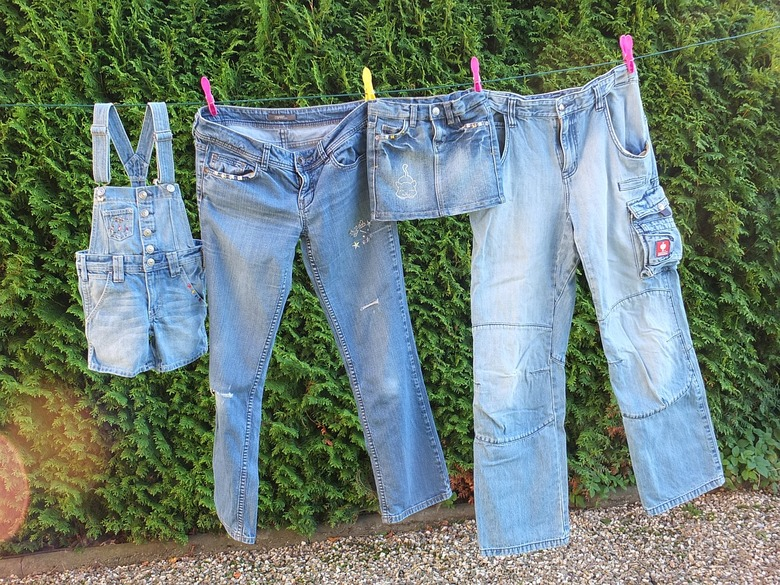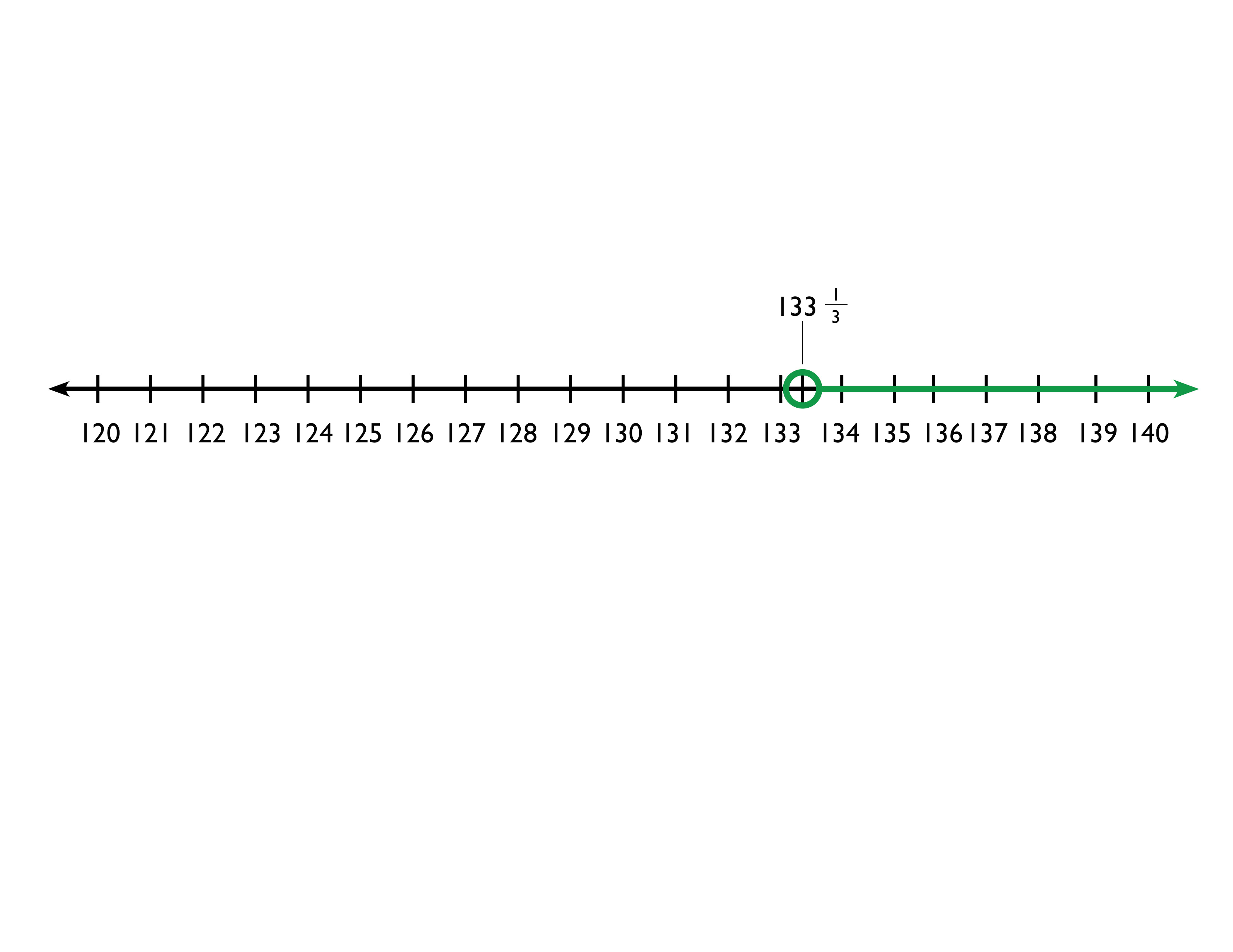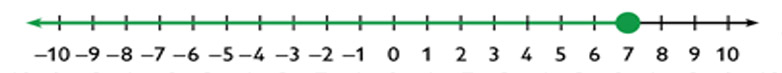Subject:
Algebra
Material Type:
Lesson Plan
Level:
Middle School
7
Provider:
Pearson
Tags:
• Inequalities
• Problem Solving
Language:
English
Media Formats:
Text/HTML

# Interpreting The Solution To An Algebraic Inequality## Overview

Students use inequalities to solve real-world problems. They see that the solution of the algebraic inequality may differ from the solution to the problem it represents. For example, a fractional number or a negative number may not be an appropriate solution for a word problem.

Students complete a Self Check. They are given an algebraic inequality that they need to solve. They then write and solve a word problem that the inequality could represent.

# Key Concepts

In this lesson, students write and solve an algebraic inequality that matches a situation given in a word problem. They then interpret that algebraic solution in the context of the problem. For example, students write and solve an algebraic inequality to represent the number of T-shirts that can be bought given a certain amount of money and another purchase. The inequality produces the solution t < 2.5. Since a fractional part of a T-shirt does not make sense, students reason that 2 is the greatest number of T-shirts that can be purchased.

# Goals and Learning Objectives

• Interpret the solution to an algebraic inequality within the context of a word problem.

# Lesson Guide

Make sure that you are familiar with the problem-solving process prior to discussing the problem-solving process with the class. You may want to post the steps in your classroom.

# Mathematics

Steps of the problem-solving process:

• Understand the problem.
• Represent the problem.

# Problem-Solving Process

You have used a process to find solutions to real-world problems that you solve with equations or formulas. This process will also help you solve inequalities.

• Understand the problem.
• Represent the problem.

# Lesson Guide

Have students work in pairs. Point out that the question that they are answering is the number of T-shirts that Jack can buy. Discuss their answers as a class.

# Mathematics

Have each pair communicate their answer. You may want to post the answers so that the class can examine them. The correct answer is 2 T-shirts. Solving the inequality leads to the solution of t ≤ 2.5. Ask students if this answer makes sense. Ask students if the solution to the inequality is the same as the solution to the word problem.

ELL: When working with ELLs, it is important to link new concepts to students' prior knowledge and experiences. This allows students to make more meaningful connections with the new mathematical concepts being learned. Explicitly teach students that since you cannot buy half a shirt, the correct answer must be 2 T-shirts.

# How Many T-Shirts?

Jack has $40 to spend on clothes for school. He wants to buy a pair of jeans and some T-shirts. The jeans cost$35 and the T-shirts cost $2. Jack writes this inequality to determine how many T-shirts he can buy. 35 + 2t ≤ 40 2t ≤ 5 • Use the inequality to determine the greatest number of T-shirts Jack can buy.# Math Mission # Lesson Guide Discuss the Math Mission. Students will write inequalities to represent real-world situations, and interpret the solutions in terms of the problem situation. ## Opening Write inequalities to represent real-world situations, and interpret the solutions in terms of the problem situation. # Memory Cards # Lesson Guide Have students work in pairs. Make sure that students know how to graph their solution. # Mathematical Practices Mathematical Practice 2: Reason abstractly and quantitatively. When writing the inequality and interpreting the solution, students are reasoning quantitatively. When solving the algebraic inequality, they are reasoning abstractly. In this lesson, they need to move between the two. # Interventions Student has difficulty getting started. • What are you trying to find? • What information do you have? • How can you estimate your answer? Student wants to include 3 as part of the solution. • Is 3 part of the solution to the inequality? • What is the total cost of 3 cards? • Is the solution to the inequality the same as the solution to the problem? [common error] Students may switch the two sides of the inequality, but not switch the direction of the inequality sign. • Ask them what happens with the numbers. Use as an example 3 < 7, but 7 > 3. # Possible Answers • Let c equal the number of cards Marcus can order. $\begin{array}{c}15.99c+5.50<50\\ 15.99c+5.50-5.50<50-5.50\\ 15.99c<44.50\\ \frac{15.99c}{15.99}<\frac{44.50}{15.99}\\ c<2.7829893...\end{array}$ • Marcus can buy 1 or 2 cards for under$50. Since Marcus cannot buy a fraction of a card, the solution is represented as x ≤ 2 on the number line.
•# Memory Cards

Marcus wants to order memory cards for his computer from an online store. If the cards cost $15.99 each, and the cost of shipping is$5.50, how many cards can he order for under $50? • Solve the inequality. • Interpret the solution to inequality. • Graph the solution to the problem on the number line. HANDOUT: Memory Cards INTERACTIVE: Graph an Inequality ## Hint: Think about what the solution to the inequality should look like based on the problem situation and question. # Water Tank # Lesson Guide Have students work in pairs as in Task 4. # Mathematical Practices Mathematical Practice 6: Attend to precision. • Students use precision when they define the variable. In this problem, they should define m as the number of minutes the water has been draining, rather than as the time the water has been draining. # Interventions Student has difficulty getting started. • What are you trying to find? • What information do you have? • How can you estimate your answer? Student writes the inequality as 1,000 + 6m < 200. • Is the water flowing in or out of the tank? • After 1 minute, will there be more or less than 1,000 gallons in the tank? Student wants to include only whole numbers in the solution. • What are you trying to find out? • Does a fraction of a minute make sense? [common error] Students may switch the two sides of the inequality, but not switch the direction of the inequality sign. • Ask them what happens with the numbers. Use as an example 3 < 7, but 7 > 3. # Possible Answers • Let m equal the number of minutes the tank has been draining. $\begin{array}{c}1,000-6m<200\\ 1,000-6m+6<200+6m\\ 1,000<200+6m\\ 1,000-200<200-200+6m\\ 800<6m\\ \frac{800}{6}<\frac{6m}{6}\\ 133\frac{1}{3}133\frac{1}{3}\end{array}$ • The tank has been draining for more than $133\frac{1}{3}$ minutes. •Some students might also realize that the water tank cannot drain indefinitely because it will be empty after a certain amount of time. They may have solved a separate inequality for this: 1,000 − 6m > 0, which would give a graph showing $133\frac{1}{3}$ < x < $166\frac{2}{3}$. ## Work Time # Water Tank A water tank contains 1,000 gallons of water. Water begins draining out of the tank at a rate of 6 gallons per minute. After a while, the tank contains less than 200 gallons of water. How long has water been draining out of the tank? • Solve the inequality. • Interpret the solution to inequality. • Graph the solution to the problem on the number line. HANDOUT: Water Tank INTERACTIVE: Graph an Inequality ## Hint: Think about what the solution to the inequality should look like based on the problem situation and question. # Prepare a Presentation # Preparing for Ways of Thinking Notice if students are following the steps for each problem. Look for students who do not understand that the solution of the inequality may not be the answer to the word problem. As you are listening to students make sure to ask them if their answers make sense. Be sure to include this point in the Ways of Thinking discussion. Look for different ways students solve the inequalities. Some students may end up with the variable on the right and some with the variable on the left. Be sure students understand that if the sides of the inequality are switched, the sign of the inequality must be reversed. ELL: Provide ELLs with clarification as to what the question asks them to do. They are asked to write an inequality, solve for a missing variable, and find a reasonable solution. # Challenge Problem ## Answer • Answers will vary. ## Work Time # Prepare a Presentation • Choose one of the problems from the previous tasks. • Show all the steps to solve your inequality. Then explain how you determined the solution to the situation and how you graphed that on the number line. # Challenge Problem • Write an explanation of the steps you use to solve an inequality, including how to interpret the solution based on the problem situation. # Make Connections # Mathematics Facilitate the discussion to help students understand the mathematics of the lesson informally. Ask questions such as the following: • What should the solution to the Memory Cards problem look like based on the information that you are given? • What should the solution to the Water Tank problem look like based on the problem situation? • Why did you use the “less than or equal to symbol” in the Memory Cards problem? • Why did you use the “less than” symbol in the Water Tank problem? • Did you reverse the inequality sign? Why? • Could −1 be a solution to the Memory Card problem? Why? • For the Water Tank problem, does the answer include the number of minutes for which there are exactly 200 gallons of water in the tank? Why? SWD: One way to start the discussion is to ask students what questions arose during the Memory Cards and Water Tank problems. Student-initiated inquiry promotes connection and engagement with the mathematics. Provide positive feedback, using gestures and prompting to elicit deeper responses. ## Performance Task # Ways of Thinking: Make Connections Take notes about other classmates' solutions, and their interpretations of the solutions. ## Hint: As your classmates present, ask questions such as: • Why did you use the “less than” symbol instead of the “less than or equal to” symbol? • Why did you reverse the inequality sign? • Why didn’t you reverse the inequality sign? • Why isn’t “–1” a solution to the problem? # Inequalities # A Possible Summary Algebraic inequalities can be used to solve word problems. However, you need to understand what the problem is about in order to use the solution of the inequality to solve the problem. For example, it does not make sense to have a fraction of a T-shirt. You cannot buy a negative number of items. It is important to always look at the answer to a word problem in terms of the context of the problem. ## Formative Assessment # Summary of the Math: Inequalities Write a summary about solving inequalities and interpreting solutions. ## Hint: Check your summary: • Do you explain how to graph an inequality on a number line? • Do you explain how to interpret a solution, and discuss the types of factors in a problem that might require you to adjust the mathematical solution to fit the problem situation? # Working With Inequalities # Lesson Guide This task allows you to assess students’ work and determine what difficulties they are having. The results of the Self Check will help you determine which students should work on the Gallery and which students would benefit from review before the assessment. Have students work on the Self Check individually. Remind students to take a screen capture of their interactive work and save to their Notebook. Students will share this work with you and receive feedback in the next lesson. # Assessment Have students submit their work to you. Make notes on what their work reveals about their current levels of understanding and their different problem-solving approaches. Do not score students’ work. Share with each student the most appropriate Interventions to guide their thought process. Also note students with a particular issue so that you can work with them in the Putting It Together lesson that follows. # Interventions Student makes errors when solving the inequality. • When do you reverse the direction of an inequality sign? • Do you have the variable by itself on one side of the inequality sign? Student does not graph the solution correctly. • Is your graph going in the correct direction? • Should you have an open or a closed circle? • Use your graph to find one number that should make the inequality true and one that should make it false. Substitute those numbers into the original inequality and see what happens. Student does not include a word problem. • Try one of these scenarios: • You want to spend no more than$66 at the store.…
• You have a piece of ribbon 66 inches long.…

Student's word problem does not match the inequality given.

• Work backward. Read the word problem you wrote. Write an inequality that represents it. Does it match the inequality you were given?

Student does not know how to connect the solution of the inequality to the solution to the word problem.

• Will all numbers on the solution graph make sense as solutions to your problem?

1. $\begin{array}{c}8x+10\le 66\\ 8x+10-10\le 66-10\\ 8x\le 56\\ \frac{8x}{8}\le \frac{56}{8}\\ x\le 7\end{array}$

x is less than or equal to 72. Sample word problem: A bucket of balls weighs 66 ounces or less. If the bucket, without any balls, weighs 10 ounces and each ball weighs 8 ounces, how many balls could be in the bucket?

3. There could be any whole number of balls from 0 to 7.

# Working With Inequalities

Complete this Self Check by yourself.

1. Solve the inequality (show all your steps) and represent the solution on the given number line.
8x + 10 ≤ 66
2. Write a word problem that could be solved using the inequality.

HANDOUT: Solving and Checking Inequalities
INTERACTIVE: Graphing an Inequality